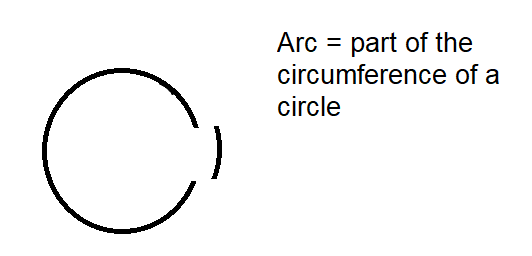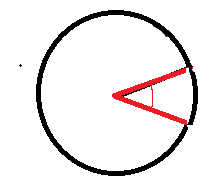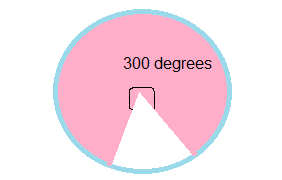# Calculating Parts of a Circle

## Arc LengthsIt’s like working out the amount of crust on your slice of pizza!

To work out the length of an arc we need to remember that it is simply a fraction of the circle.

The fraction of the arc can be calculated by dividing the angle of the two radii between the arc by 360.

This formula can be used to work out the length of an arc:

= Angle/360 x circumference

= Angle/360 x π x diameter

Eg. If I have an arc that is enclosed by an angle of 20 degrees and my diameter is 4 cm the arc length

= 20/360 x π x 4

= 0.698 cm (to 3 dp)

## Area of sectors

To work out the area of a sector I can use a similar method. As I want a fraction of the area of the circle.

=Angle/360 x area of a circle

=Angle/360 x π x r x r

For example I want to work out the area of the pink sector of this circle that has a radius of 5 cm I simply use the formula:= Angle/360 x π x r x r

= 300/360 x π x 5 x 5

= 65.45 cm2 (to 2 dp)

## Angles of sectors

We can also reverse the above formulas to work out the angles of sectors.

For example, if I have a sector with an area of 5 cm2 and a radius of 3 cm I can work out the angle of the sector.

Start by using the original formula and inputting the information that you know:

5 = Angle/360 x π x 3 x 3

5 = Angle/360 x π x 9

5/9π = Angle/360

5/9π x 360 = 64.29

Angle = 64.29 (to 2 dp)